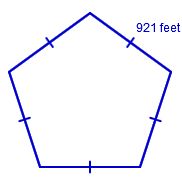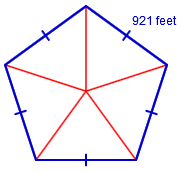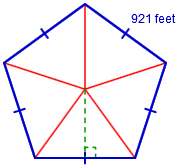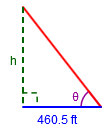### Home > INT2 > Chapter 9 > Lesson 9.3.1 > Problem9-89

9-89.

The United State Department of Defense is located in a building called the Pentagon because it is in the shape of a regular pentagon. Known as “the largest office building in the world,” its exterior edges measure $921$ feet.

1. What is the area of land enclosed by the outer walls of the Pentagon building?

1. If 1 mile is equal to $5280$ feet, what is that area in square miles?Find the area using trigonometric ratios.The interior angles of a regular pentagon measure  $\frac{180(5-2)}{5}=\frac{180\cdot3}{5}=\frac{540}{5}=108^\circ$

$\Theta = \frac{108^\circ}{2}$